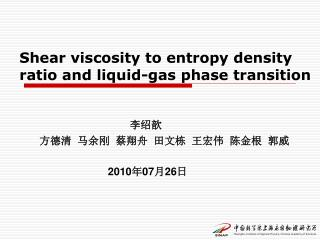DownloadDownload PresentationShear viscosity to entropy density ratio and liquid-gas phase transition

# Shear viscosity to entropy density ratio and liquid-gas phase transition

Download Presentation## Shear viscosity to entropy density ratio and liquid-gas phase transition

- - - - - - - - - - - - - - - - - - - - - - - - - - - E N D - - - - - - - - - - - - - - - - - - - - - - - - - - -
##### Presentation Transcript

1. Shear viscosity to entropy density ratio and liquid-gas phase transition 李绍歆 方德清 马余刚 蔡翔舟 田文栋 王宏伟 陈金根 郭威 2010年07月26日

2. outline • Introduction viscosityand liquid-gas transition BUU Model • our work viscosity in intermediate energy heavy ion collisions the lowest value of viscosity/entropy? • Summary and Conclusions

3. motivation • In ultra relativistic heavy ion collision, we often study the QGP phase and critical phenomenon according to the minimum value of viscosity/entropy. • Due to van der Waals nature of the interaction forceof nucleon-nucleon, Liquid-gas transition happen in intermediate heavy-ion collisions. • the lowest value of viscosity/entropy can be a signal of liquid-gas phase transition phase in nuclear reaction? arXiv:0812.2422

4. 我们的猜测：核液气相变（红虚线是我们手画的线）：YGM, 云南会议2007 Perfect liquid? Lacey et al., PRL 98, 092301 (2007)‏

5. BUU model • Description of the model. 1) Mean field 2) Two-body collisions 3) Pauli blocking

6. Green-kubo formula • The Green-Kubo formalism relates linear transport coefficients to near-equilibrium correlations of dissipative fluxes . where if the nucleons are uniformly distributed inspace Here t=0 is equilibrium time If volume is fixed PHYSICAL REVIEW C 69, 044901 (2004)‏

7. temperature • Au+Au 50~100MeV r=5fm • extract phase space information • Boltzmann distribution t=70 fm/c n/n0=1 (n0=0.16) t=100fm/c n/n0=1/2 t=140fm/c n/n0=1/4 Where is the energy of nuclei i 高能段拟合上

8. Equilibrium Au+Au 50~100MeV • Thermodynamic equilibrium( temperature approach to fixed data)‏ • Kinetic condition • So the equilibrium time t0>=100fm/c • Different energy has different t0 pk 趋于定值 t>t0 ,温度变化缓慢

9. tensor PHYSICAL REVIEW C 69, 044901 (2004)‏ 驰豫时间随入射能量逐渐减小

10. Entropy density 定体积内熵随入射能量减小 PHYSICAL REVIEW C 69, 044901 (2004)‏

11. Viscosity / entropy • Different incident beam energy(50~100MeV)‏ • Temperature (after equilibrium)‏ • conclusion: The lowest valueof eta/s between 70 and 80MeV. 最低点位置

12. Conclusion • The lowest value of viscosity/entropy between 70 and 80MeV • The other way ( power-law and LN(Amax)-S2) show Liquid-gas transition happen when the incident beam energy about 80MeV . • problem : the result is sensitivity to the equilibrium time . • Improvement: we need more incident energy data other way to calculate state parameter and eta other judgment and experiment data to prove our conclusion

13. Thanks all !